# Chapter 9 q&p

18 de Jun de 2014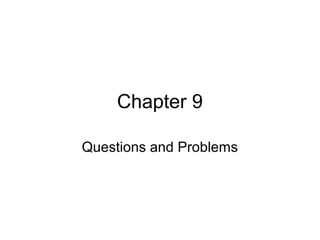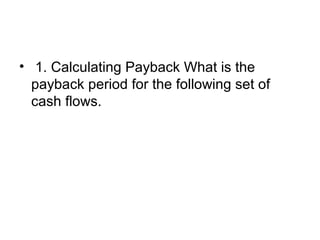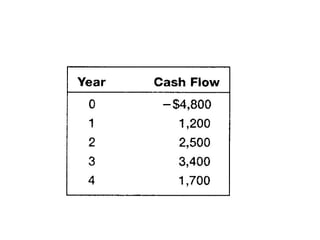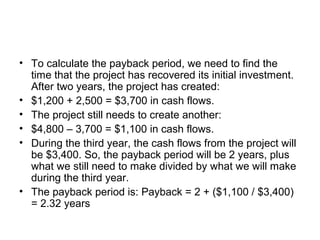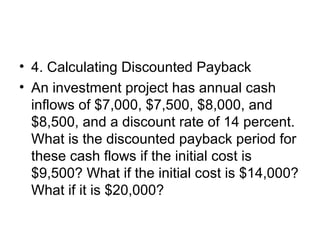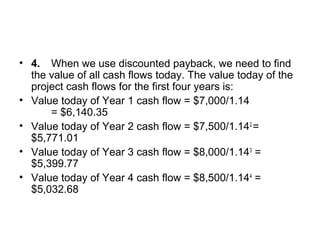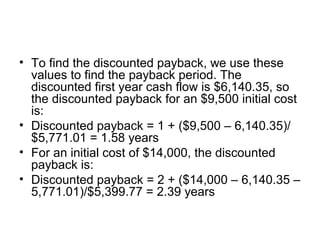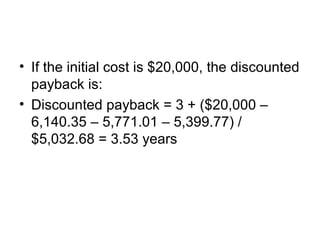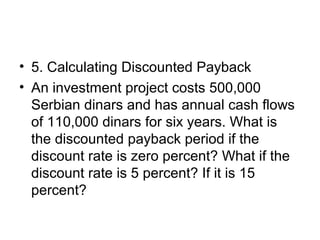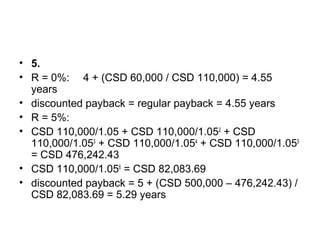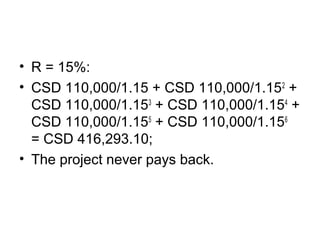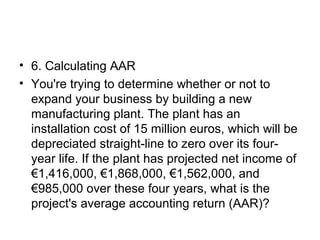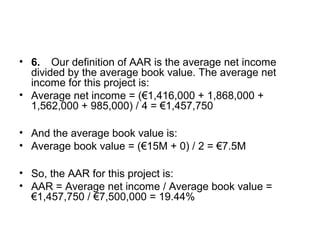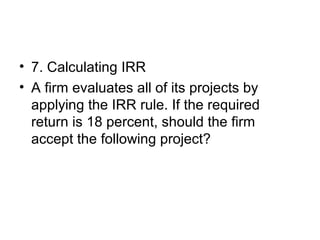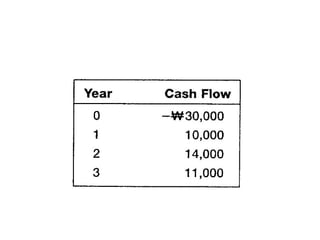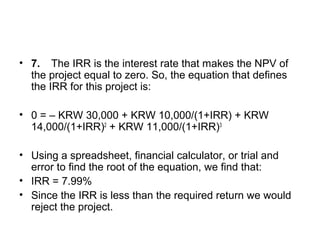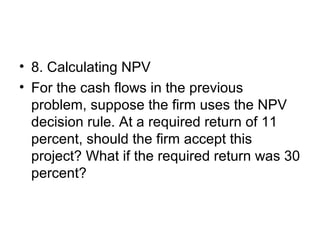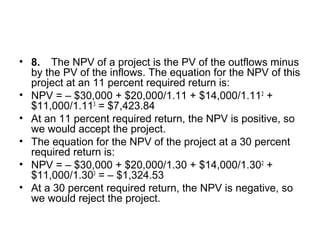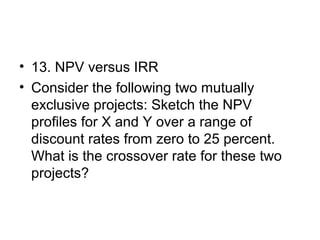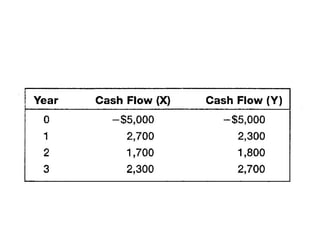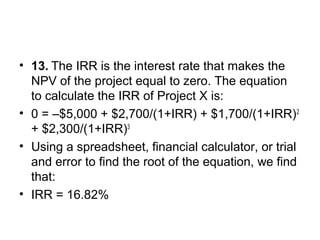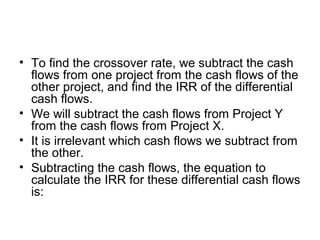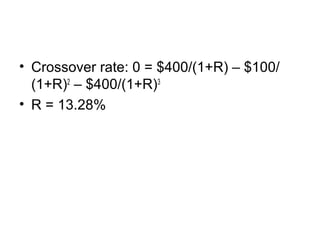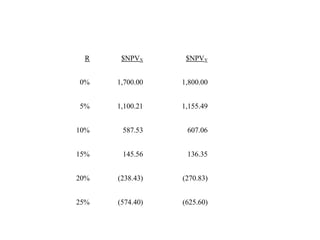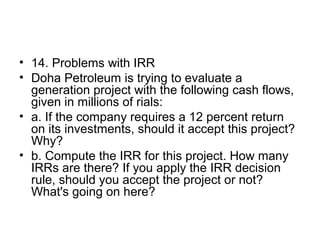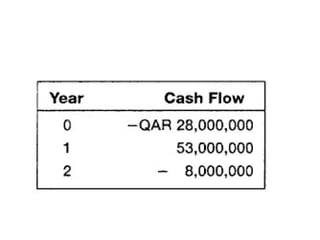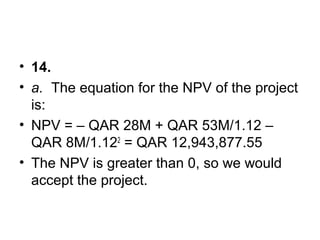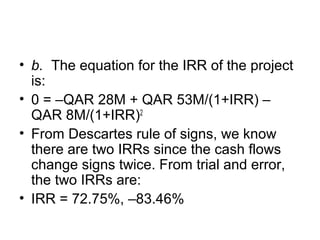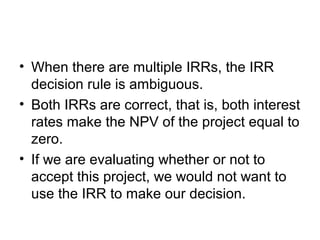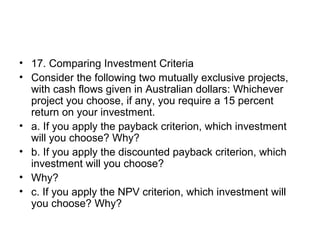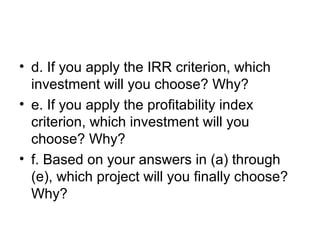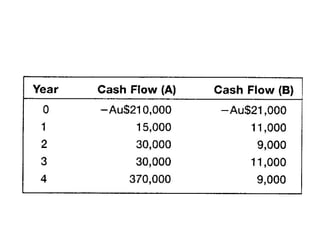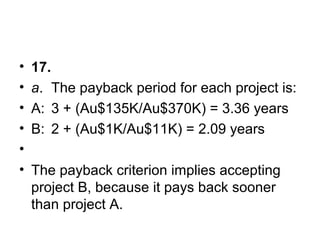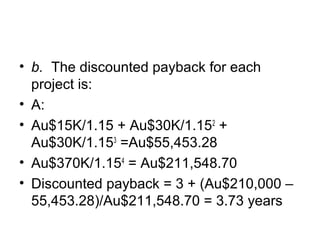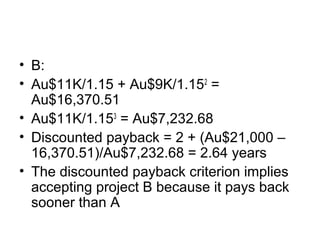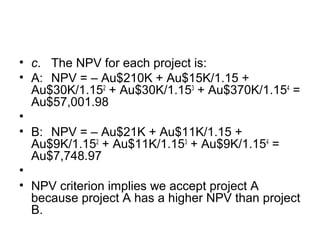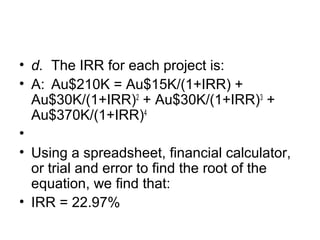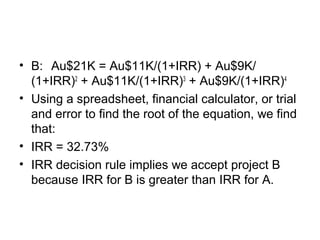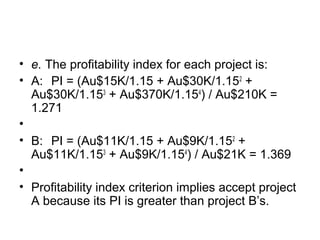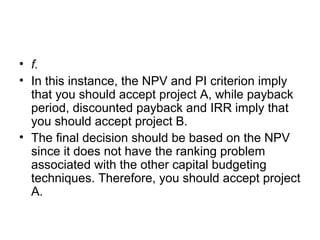1 de 41

### Chapter 9 q&p

• 2. • 1. Calculating Payback What is the payback period for the following set of cash flows.
• 4. • To calculate the payback period, we need to find the time that the project has recovered its initial investment. After two years, the project has created: • \$1,200 + 2,500 = \$3,700 in cash flows. • The project still needs to create another: • \$4,800 – 3,700 = \$1,100 in cash flows. • During the third year, the cash flows from the project will be \$3,400. So, the payback period will be 2 years, plus what we still need to make divided by what we will make during the third year. • The payback period is: Payback = 2 + (\$1,100 / \$3,400) = 2.32 years
• 5. • 4. Calculating Discounted Payback • An investment project has annual cash inflows of \$7,000, \$7,500, \$8,000, and \$8,500, and a discount rate of 14 percent. What is the discounted payback period for these cash flows if the initial cost is \$9,500? What if the initial cost is \$14,000? What if it is \$20,000?
• 6. • 4. When we use discounted payback, we need to find the value of all cash flows today. The value today of the project cash flows for the first four years is: • Value today of Year 1 cash flow = \$7,000/1.14 = \$6,140.35 • Value today of Year 2 cash flow = \$7,500/1.142 = \$5,771.01 • Value today of Year 3 cash flow = \$8,000/1.143 = \$5,399.77 • Value today of Year 4 cash flow = \$8,500/1.144 = \$5,032.68
• 7. • To find the discounted payback, we use these values to find the payback period. The discounted first year cash flow is \$6,140.35, so the discounted payback for an \$9,500 initial cost is: • Discounted payback = 1 + (\$9,500 – 6,140.35)/ \$5,771.01 = 1.58 years • For an initial cost of \$14,000, the discounted payback is: • Discounted payback = 2 + (\$14,000 – 6,140.35 – 5,771.01)/\$5,399.77 = 2.39 years
• 8. • If the initial cost is \$20,000, the discounted payback is: • Discounted payback = 3 + (\$20,000 – 6,140.35 – 5,771.01 – 5,399.77) / \$5,032.68 = 3.53 years
• 9. • 5. Calculating Discounted Payback • An investment project costs 500,000 Serbian dinars and has annual cash flows of 110,000 dinars for six years. What is the discounted payback period if the discount rate is zero percent? What if the discount rate is 5 percent? If it is 15 percent?
• 10. • 5. • R = 0%: 4 + (CSD 60,000 / CSD 110,000) = 4.55 years • discounted payback = regular payback = 4.55 years • R = 5%: • CSD 110,000/1.05 + CSD 110,000/1.052 + CSD 110,000/1.053 + CSD 110,000/1.054 + CSD 110,000/1.055 = CSD 476,242.43 • CSD 110,000/1.056 = CSD 82,083.69 • discounted payback = 5 + (CSD 500,000 – 476,242.43) / CSD 82,083.69 = 5.29 years
• 11. • R = 15%: • CSD 110,000/1.15 + CSD 110,000/1.152 + CSD 110,000/1.153 + CSD 110,000/1.154 + CSD 110,000/1.155 + CSD 110,000/1.156 = CSD 416,293.10; • The project never pays back.
• 12. • 6. Calculating AAR • You're trying to determine whether or not to expand your business by building a new manufacturing plant. The plant has an installation cost of 15 million euros, which will be depreciated straight-line to zero over its four- year life. If the plant has projected net income of €1,416,000, €1,868,000, €1,562,000, and €985,000 over these four years, what is the project's average accounting return (AAR)?
• 13. • 6. Our definition of AAR is the average net income divided by the average book value. The average net income for this project is: • Average net income = (€1,416,000 + 1,868,000 + 1,562,000 + 985,000) / 4 = €1,457,750 • And the average book value is: • Average book value = (€15M + 0) / 2 = €7.5M • So, the AAR for this project is: • AAR = Average net income / Average book value = €1,457,750 / €7,500,000 = 19.44%
• 14. • 7. Calculating IRR • A firm evaluates all of its projects by applying the IRR rule. If the required return is 18 percent, should the firm accept the following project?
• 16. • 7. The IRR is the interest rate that makes the NPV of the project equal to zero. So, the equation that defines the IRR for this project is: • 0 = – KRW 30,000 + KRW 10,000/(1+IRR) + KRW 14,000/(1+IRR)2 + KRW 11,000/(1+IRR)3 • Using a spreadsheet, financial calculator, or trial and error to find the root of the equation, we find that: • IRR = 7.99% • Since the IRR is less than the required return we would reject the project.
• 17. • 8. Calculating NPV • For the cash flows in the previous problem, suppose the firm uses the NPV decision rule. At a required return of 11 percent, should the firm accept this project? What if the required return was 30 percent?
• 18. • 8. The NPV of a project is the PV of the outflows minus by the PV of the inflows. The equation for the NPV of this project at an 11 percent required return is: • NPV = – \$30,000 + \$20,000/1.11 + \$14,000/1.112 + \$11,000/1.113 = \$7,423.84 • At an 11 percent required return, the NPV is positive, so we would accept the project. • The equation for the NPV of the project at a 30 percent required return is: • NPV = – \$30,000 + \$20,000/1.30 + \$14,000/1.302 + \$11,000/1.303 = – \$1,324.53 • At a 30 percent required return, the NPV is negative, so we would reject the project.
• 19. • 13. NPV versus IRR • Consider the following two mutually exclusive projects: Sketch the NPV profiles for X and Y over a range of discount rates from zero to 25 percent. What is the crossover rate for these two projects?
• 21. • 13. The IRR is the interest rate that makes the NPV of the project equal to zero. The equation to calculate the IRR of Project X is: • 0 = –\$5,000 + \$2,700/(1+IRR) + \$1,700/(1+IRR)2 + \$2,300/(1+IRR)3 • Using a spreadsheet, financial calculator, or trial and error to find the root of the equation, we find that: • IRR = 16.82%
• 22. • For Project Y, the equation to find the IRR is: • 0 = –\$5,000 + \$2,300/(1+IRR) + \$1,800/ (1+IRR)2 + \$2,700/(1+IRR)3 • Using a spreadsheet, financial calculator, or trial and error to find the root of the equation, we find that: • IRR = 16.60%
• 23. • To find the crossover rate, we subtract the cash flows from one project from the cash flows of the other project, and find the IRR of the differential cash flows. • We will subtract the cash flows from Project Y from the cash flows from Project X. • It is irrelevant which cash flows we subtract from the other. • Subtracting the cash flows, the equation to calculate the IRR for these differential cash flows is:
• 24. • Crossover rate: 0 = \$400/(1+R) – \$100/ (1+R)2 – \$400/(1+R)3 • R = 13.28%
• 25. R \$NPVX \$NPVY 0% 1,700.00 1,800.00 5% 1,100.21 1,155.49 10% 587.53 607.06 15% 145.56 136.35 20% (238.43) (270.83) 25% (574.40) (625.60)
• 26. • 14. Problems with IRR • Doha Petroleum is trying to evaluate a generation project with the following cash flows, given in millions of rials: • a. If the company requires a 12 percent return on its investments, should it accept this project? Why? • b. Compute the IRR for this project. How many IRRs are there? If you apply the IRR decision rule, should you accept the project or not? What's going on here?
• 28. • 14. • a. The equation for the NPV of the project is: • NPV = – QAR 28M + QAR 53M/1.12 – QAR 8M/1.122 = QAR 12,943,877.55 • The NPV is greater than 0, so we would accept the project.
• 29. • b. The equation for the IRR of the project is: • 0 = –QAR 28M + QAR 53M/(1+IRR) – QAR 8M/(1+IRR)2 • From Descartes rule of signs, we know there are two IRRs since the cash flows change signs twice. From trial and error, the two IRRs are: • IRR = 72.75%, –83.46%
• 30. • When there are multiple IRRs, the IRR decision rule is ambiguous. • Both IRRs are correct, that is, both interest rates make the NPV of the project equal to zero. • If we are evaluating whether or not to accept this project, we would not want to use the IRR to make our decision.
• 31. • 17. Comparing Investment Criteria • Consider the following two mutually exclusive projects, with cash flows given in Australian dollars: Whichever project you choose, if any, you require a 15 percent return on your investment. • a. If you apply the payback criterion, which investment will you choose? Why? • b. If you apply the discounted payback criterion, which investment will you choose? • Why? • c. If you apply the NPV criterion, which investment will you choose? Why?
• 32. • d. If you apply the IRR criterion, which investment will you choose? Why? • e. If you apply the profitability index criterion, which investment will you choose? Why? • f. Based on your answers in (a) through (e), which project will you finally choose? Why?
• 34. • 17. • a. The payback period for each project is: • A: 3 + (Au\$135K/Au\$370K) = 3.36 years • B: 2 + (Au\$1K/Au\$11K) = 2.09 years • • The payback criterion implies accepting project B, because it pays back sooner than project A.
• 35. • b. The discounted payback for each project is: • A: • Au\$15K/1.15 + Au\$30K/1.152 + Au\$30K/1.153 =Au\$55,453.28 • Au\$370K/1.154 = Au\$211,548.70 • Discounted payback = 3 + (Au\$210,000 – 55,453.28)/Au\$211,548.70 = 3.73 years
• 36. • B: • Au\$11K/1.15 + Au\$9K/1.152 = Au\$16,370.51 • Au\$11K/1.153 = Au\$7,232.68 • Discounted payback = 2 + (Au\$21,000 – 16,370.51)/Au\$7,232.68 = 2.64 years • The discounted payback criterion implies accepting project B because it pays back sooner than A
• 37. • c. The NPV for each project is: • A: NPV = – Au\$210K + Au\$15K/1.15 + Au\$30K/1.152 + Au\$30K/1.153 + Au\$370K/1.154 = Au\$57,001.98 • • B: NPV = – Au\$21K + Au\$11K/1.15 + Au\$9K/1.152 + Au\$11K/1.153 + Au\$9K/1.154 = Au\$7,748.97 • • NPV criterion implies we accept project A because project A has a higher NPV than project B.
• 38. • d. The IRR for each project is: • A: Au\$210K = Au\$15K/(1+IRR) + Au\$30K/(1+IRR)2 + Au\$30K/(1+IRR)3 + Au\$370K/(1+IRR)4 • • Using a spreadsheet, financial calculator, or trial and error to find the root of the equation, we find that: • IRR = 22.97%
• 39. • B: Au\$21K = Au\$11K/(1+IRR) + Au\$9K/ (1+IRR)2 + Au\$11K/(1+IRR)3 + Au\$9K/(1+IRR)4 • Using a spreadsheet, financial calculator, or trial and error to find the root of the equation, we find that: • IRR = 32.73% • IRR decision rule implies we accept project B because IRR for B is greater than IRR for A.
• 40. • e. The profitability index for each project is: • A: PI = (Au\$15K/1.15 + Au\$30K/1.152 + Au\$30K/1.153 + Au\$370K/1.154 ) / Au\$210K = 1.271 • • B: PI = (Au\$11K/1.15 + Au\$9K/1.152 + Au\$11K/1.153 + Au\$9K/1.154 ) / Au\$21K = 1.369 • • Profitability index criterion implies accept project A because its PI is greater than project B’s.
• 41. • f. • In this instance, the NPV and PI criterion imply that you should accept project A, while payback period, discounted payback and IRR imply that you should accept project B. • The final decision should be based on the NPV since it does not have the ranking problem associated with the other capital budgeting techniques. Therefore, you should accept project A.